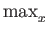Next: Nondeterministically Evasive Functions Up: Boolean Functions Previous: Tree Functions   Contents

# Nondeterministic Decision Tree Complexity

In the presentation of the most general results of this thesis we refer frequently to deterministic and nondeterministic decision tree complexity, deterministic decision trees were discussed in Section 3.1. Readers familiar with these topics can proceed directly to Section 4.3.

Let f be an N-bit Boolean function. We will use the following definitions of László Lovász and Péter Gács .

For every input x let D(f, x) denote the minimum number of bits of x we could be told to convince us that f (x) takes on a particular value. For example: D(OR , 0N) = N. If we are told fewer than N bits of an input are all 0 we can not determine the value of OR for that input. For any other input x0N, D(OR, x) = 1, because we only need to be told that one bit of the input is a 1. We are not concerned with how many oracle queries we have to make in the worst case to determine f (x), but rather how many bits we could be told in the best case to be sure of the value f takes on x.

Definition 4.2.1   D0(f )= max{D(f, x)| f (x) = 0}. D0(f ) is the nondeterministic decision tree complexity of verifying that an input takes on 0 when evaluated by f.

Definition 4.2.2   D1(f )= max{D(f, x)| f (x) = 1}. D1(f ) is the nondeterministic decision tree complexity of verifying that an input takes on 1 when evaluated by f.

Definition 4.2.3   N(f )= max{D0(f ), D1(f )} ={D(f, x)}. The nondeterministic decision tree complexity of computing an N-bit Boolean function f is the maximum of the nondeterministic decision tree complexities of verifying any input of f takes on one of {0, 1}.Next: Nondeterministically Evasive Functions Up: Boolean Functions Previous: Tree Functions   Contents
Matthew Hayward Lower Query Bounds in the Quantum Oracle Model GitHub Repository